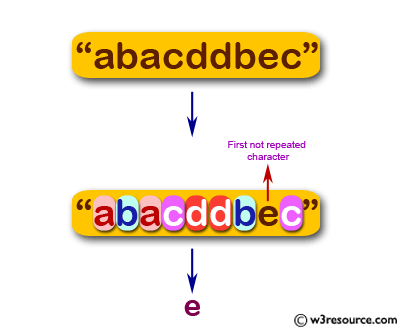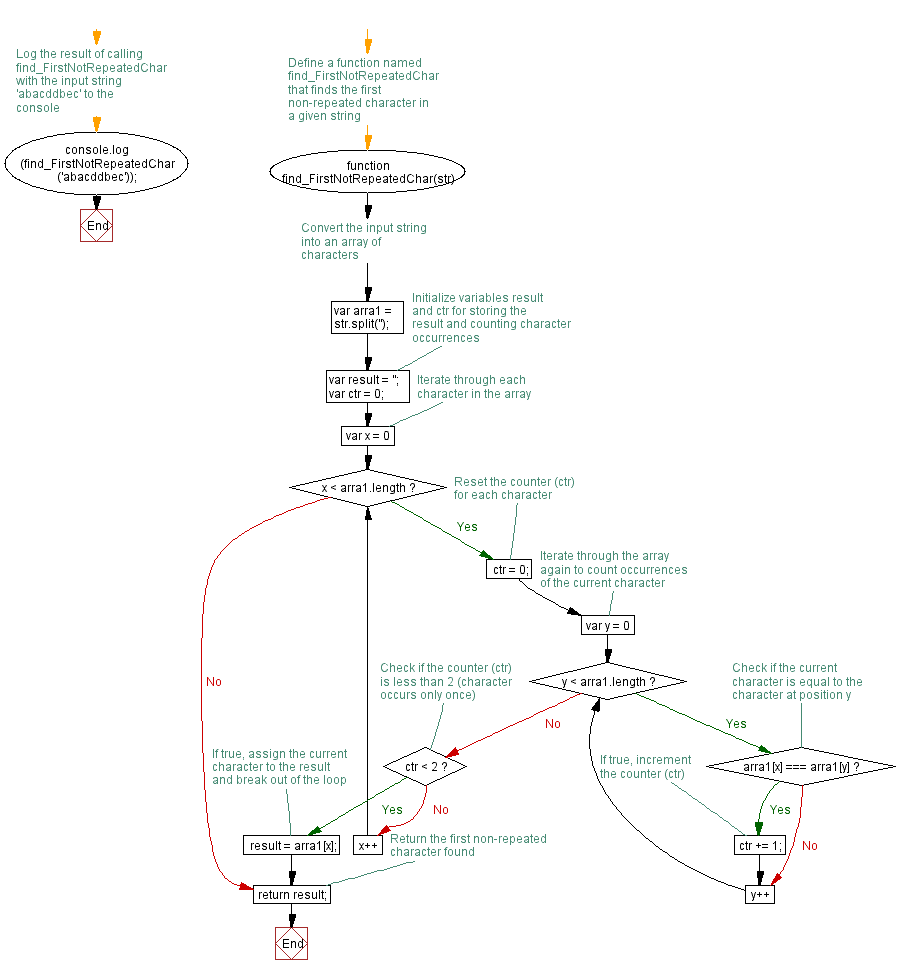JavaScript: Find the first not repeated character

JavaScript Function: Exercise-23 with Solution

Write a JavaScript function to find the first not repeated character.

Sample arguments : 'abacddbec'
Expected output : 'e'

Pictorial Presentation:Sample Solution: -

HTML Code:

<!DOCTYPE html>
<html>
<meta charset="utf-8">
<title>find the first not repeated character.</title>
<body>

</body>
</html>

JavaScript Code:

function find_FirstNotRepeatedChar(str) {
var arra1 = str.split('');
var result = '';
var ctr = 0;

for (var x = 0; x < arra1.length; x++) {
ctr = 0;

for (var y = 0; y < arra1.length; y++)
{
if (arra1[x] === arra1[y]) {
ctr+= 1;
}
}

if (ctr < 2) {
result = arra1[x];
break;
}
}
return result;
}
console.log(find_FirstNotRepeatedChar('abacddbec'));

Sample Output:

e

Flowchart:Live Demo:

See the Pen JavaScript - Find the first not repeated character-function-ex- 23 by w3resource (@w3resource) on CodePen.

Improve this sample solution and post your code through Disqus

What is the difficulty level of this exercise?

﻿